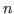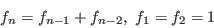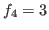: Implementing a Java-like language : Using sm1 to teach : Using sm1 to teach   目次

## Recursive call and the stack

The notion of stack is one of the most important idea in computer science. The notion of recursive call of functions is usually taught in the first course of programming. I think it is important to understand how the stack is used to emulate recurisve calls. The idea is the use of the stack. Function arguments and local variables are stored in the stack. It enables the system to restore the values of the local variables and arguments after an execution of the function. However, it should be noted that, for each function call, the stack dynamically grows.

As an example that I used in a class room, let us evaluate the-th Fibonacci number defined byby using a recurisive call.
  /fib {
/arg1 set
[/n /ans] pushVariables
pstack
/n arg1 def
(n=) messagen n message
(-------------------------------) message
n 2 le {
/ans  1  def
}
{
n 1 sub fib  n 2 sub fib add /ans set
} ifelse
/arg1 ans def
popVariables
arg1
} def

The program would return the-th Fibonacci number. That is,  4 fib ::  would return. It also output the entire stack at each call, so you can observe how stack grows during the computation and how local variables n, ans are stored in the stack. You would also realize that this program is not efficient and exhausts huge memory space.: Implementing a Java-like language : Using sm1 to teach : Using sm1 to teach   目次
Nobuki Takayama 平成22年2月8日